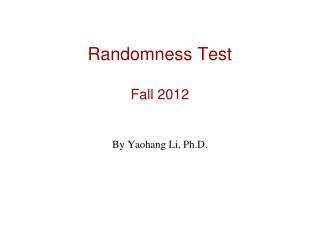Download PresentationRandomness Test Fall 2012

# Randomness Test Fall 2012

Download Presentation## Randomness Test Fall 2012

- - - - - - - - - - - - - - - - - - - - - - - - - - - E N D - - - - - - - - - - - - - - - - - - - - - - - - - - -
##### Presentation Transcript

1. Randomness TestFall 2012 By Yaohang Li, Ph.D.

2. Review • Last Class • Random Number Generation • Uniform Distribution • This Class • Test of Randomness • Chi Square Test • K-S Test • 10 empirical tests • Next Class • Nuclear Simulation

3. Chi-square test • Introduced by Karl Pearson in 1900 • Test for discrete distributions e.g. binomial and Poisson distributions • Implementation: - assume we have k possible categories - P = sequence size / k - expected sample size = P * n trials - suppose category i occurs Yi times - error = Yi – nPi - chi-square statistic - chi-square percentile = proportion of samples from a "true“ distribution having a chi-square statistic (function of errors) less than the percentile.

4. Example • Given two “true” dice for 144 trials we get: • s = 2 3 4 5 6 7 8 9 10 11 12 • Ps = 1/36 1/18 1/12 1/9 5/36 1/6 5/36 1/9 1/12 1/18 1/36 • Ys = 2 4 10 12 22 29 21 15 14 9 6 • nPs = 4 8 12 16 20 24 20 16 12 8 4 • V = (Y2 – nP2)² / nP2 + (Y3 – nP3)² / nP3 +………+ (Y12 – nP12)² / nP12 • V = (2 – 4)² / 4 + (4 – 8)² / 8 +……+ (9 – 8)² / 8 + (6 – 4)² / 4 = 7 7/48

5. Chi-square Table

6. Kolmogorov-Smirnov test • Introduced in 1933 • Test for continuous distributions e.g. normal and Weibull distributions • based on ECDF defined as,

7. K-S Test

8. K-S Table

9. Empirical Tests Equidistribution Test Serial Test Gap Test Poker Test Coupon Collector’s Test Permutation Test Run Test Maximum of t test Collision Test Serial Correlation Test

10. Summary • Chi-Square Test • KS Test • Empirical Tests

11. What I want you to do? • Review Slides • Review basic probability/statistics concepts • Work on your Assignment 3